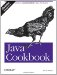# Recipe 5.19 Handling Very Large Numbers

#### Problem

You need to handle integer numbers larger than Long.MAX_VALUE or floating-point values larger than Double.MAX_VALUE.

#### Solution

Use the BigInteger or BigDecimal values in package java.math :

`// BigNums.java  System.out.println("Here's Long.MAX_VALUE: " + Long.MAX_VALUE);  BigInteger bInt = new BigInteger("3419229223372036854775807");  System.out.println("Here's a bigger number: " + bInt);  System.out.println("Here it is as a double: " + bInt.doubleValue( ));`

Note that the constructor takes the number as a string. Obviously you couldn't just type the numeric digits since by definition these classes are designed to represent numbers larger than will fit in a Java long.

#### Discussion

Both BigInteger and BigDecimal objects are immutable; that is, once constructed, they always represent a given number. That said, a number of methods return new objects that are mutations of the original, such as negate( ) , which returns the negative of the given BigInteger or BigDecimal. There are also methods corresponding to most of the Java language built-in operators defined on the base types int/long and float/double. The division method makes you specify the rounding method; consult a book on numerical analysis for details. Example 5-5 is a simple stack-based calculator using BigDecimal as its numeric data type.

##### Example 5-5. BigNumCalc
`import java.math.BigDecimal; import java.util.Stack; /** A trivial reverse-polish stack-based calculator for big numbers */ public class BigNumCalc {     /** an array of Objects, simulating user input */     public static Object[] testInput = {         new BigDecimal("3419229223372036854775807.23343"),         new BigDecimal("2.0"),         "*",     };     public static void main(String[] args) {         BigNumCalc calc = new BigNumCalc( );         System.out.println(calc.calculate(testInput));     }     Stack s = new Stack( );     public BigDecimal calculate(Object[] input) {         BigDecimal tmp;         for (int i = 0; i < input.length; i++) {             Object o = input[i];             if (o instanceof BigDecimal)                 s.push(o);             else if (o instanceof String) {                 switch (((String)o).charAt(0)) {                 // + and * are commutative, order doesn't matter                 case '+':                     s.push(((BigDecimal)s.pop( )).add((BigDecimal)s.pop( )));                     break;                 case '*':                     s.push(((BigDecimal)s.pop( )).multiply((BigDecimal)s.pop( )));                     break;                 // - and /, order *does* matter                 case '-':                     tmp = (BigDecimal)s.pop( );                     s.push(((BigDecimal)s.pop( )).subtract(tmp));                     break;                 case '/':                     tmp = (BigDecimal)s.pop( );                     s.push(((BigDecimal)s.pop( )).divide(tmp,                         BigDecimal.ROUND_UP));                     break;                 default:                     throw new IllegalStateException("Unknown OPERATOR popped");                 }             } else {                 throw new IllegalStateException("Syntax error in input");             }         }         return (BigDecimal)s.pop( );     } }`

Running this produces the expected (very large) value:

`> jikes +E -d . BigNumCalc.java > java BigNumCalc 6838458446744073709551614.466860 >`

The current version has its inputs hard-coded, as does the JUnit test program, but in real life you can use regular expressions to extract words or operators from an input stream (as in Recipe 4.5), or you can use the StreamTokenizer approach of the simple calculator (Recipe Recipe 10.4). The stack of numbers is maintained using a java.util.Stack (Recipe 7.14).

BigInteger is mainly useful in cryptographic and security applications. Its method isProbablyPrime( ) can create prime pairs for public key cryptography. BigDecimal might also be useful in computing the size of the universe.Java Cookbook, Second Edition
ISBN: 0596007019
EAN: 2147483647
Year: 2003
Pages: 409
Authors: Ian F Darwin

Similar book on Amazon